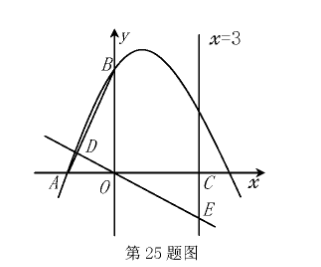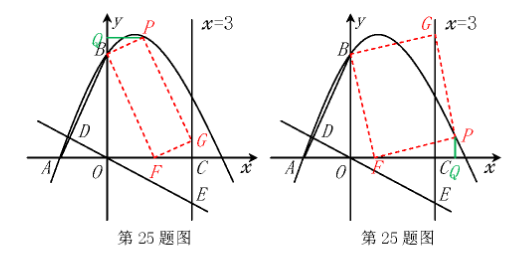(1) 求 $\mathrm{a}, \mathrm{c}$ 的值;
(2) 经过点 0 的直线分别与线段 $A B$, 直线 $x=3$ 交于点 $D, E$, 且 $\triangle B D O$ 与 $\triangle O C E$ 的面 积相等, 求直线 DE 的解析式;
(3) $\mathrm{P}$ 是抛物线上位于第一象限的一个动点, 在线段 $\mathrm{OC}$ 和直线 $x=3$ 上是否分别存在 点 $F, G$, 使 $B, F, G, P$ 为顶点的四边形是以 $B F$ 为一边的矩形? 若存在, 求出点 $F$ 的坐标; 若不存在, 请说明理由.【答案】(2) 由 $\mathrm{A}(-2,0), \mathrm{B}(0,4)$ 易知直线 $\mathrm{AB}$ 的解析式为 $\mathrm{y}=2 x+4$, 设直线 $\mathrm{DE}$ 的解析式为 $\mathrm{y}=\mathrm{k} x(\mathrm{k} < 0)$,

$\mathrm{S}_{\triangle B D 0}=\frac{1}{2} \times \mathrm{OB} \times\left|x_D\right|=\frac{1}{2} \times 4 \times\left|\frac{4}{\mathrm{k}-2}\right|=\frac{8}{2-\mathrm{k}}$
$\because \mathrm{S}_{\triangle \triangle C \mathrm{C}}=\mathrm{S}_{\triangle B B}, \therefore-\frac{9}{2} \mathrm{k}=\frac{8}{2-\mathrm{k}}$, 解得 $\mathrm{k}_1=\frac{8}{3}$ (舍去), $\mathrm{k}_2=-\frac{2}{3}$

(3) 由 (1) 知抛物线解析式为 $\mathrm{y}=-\frac{1}{2} x^2+x+4$, 设 $\mathrm{P}$ 点坐标为 $\left(\mathrm{t},-\frac{1}{2} \mathrm{t}^2+\mathrm{t}+4\right)(\mathrm{t} > 0)$
(1) 当 $P$ 在直线 $x=3$ 左侧, 即 $0 < t < 3$ 时, 过 $P$ 作 $P Q \perp y$ 轴于 $Q$,
$\because$ 四边形 BFGP 为矩形, $\therefore x_{\mathrm{B}}+x_{\mathrm{C}}=x_{\mathrm{P}}+x_{\mathrm{F}}$, 即 $3=\mathrm{t}+x_{\mathrm{F}}, \therefore x_{\mathrm{P}}=3-\mathrm{t}$,
$\because \angle \mathrm{PQB}=\angle \mathrm{PBF}=90^{\circ}, \quad \therefore \angle \mathrm{OBF}+\angle \mathrm{PBQ}=\angle \mathrm{PBQ}+\angle \mathrm{QPB}=90^{\circ}, \therefore \angle \mathrm{OBF}=\angle \mathrm{QPB}$, 又 $\because \angle \mathrm{PQB}=$
$\angle \mathrm{BOF}=90^{\circ}, \therefore \triangle \mathrm{PQB} \sim \triangle \mathrm{BOF}, \therefore \frac{\mathrm{OF}}{\mathrm{BQ}}=\frac{\mathrm{OB}}{\mathrm{PQ}}$, 即 $\frac{x_{\mathrm{F}}}{-\frac{1}{2} \mathrm{t}^2+\mathrm{t}}=\frac{4}{\mathrm{t}}$, 代入 $x_{\mathrm{F}}=3-\mathrm{t}$, 得 $\mathrm{t}^2-\mathrm{t}=0$, 解得 $\mathrm{t}_1=0$ (舍去), $\mathrm{t}_2=1, \therefore x_{\mathrm{P}}=3-\mathrm{t}=2$, 即 $\mathrm{F}$ 点坐标为 $(2,0)$;
(2) 当 $\mathrm{P}$ 在直线 $x=3$ 右侧, 即 $\mathrm{t} \geqslant 3$ 时, 过 $\mathrm{P}$ 作 $\mathrm{PQ} \perp x$ 轴于 $Q$,
$\because$ 四边形 $\mathrm{BFPG}$ 为矩形, $\therefore x_{\mathrm{B}}+x_{\mathrm{F}}=x_0+x_{\mathrm{F}}$, 即 $\mathrm{t}=3+x_{\mathrm{F}}, \therefore x_{\mathrm{P}}=\mathrm{t}-3, \mathrm{t}-x_{\mathrm{F}}=3$